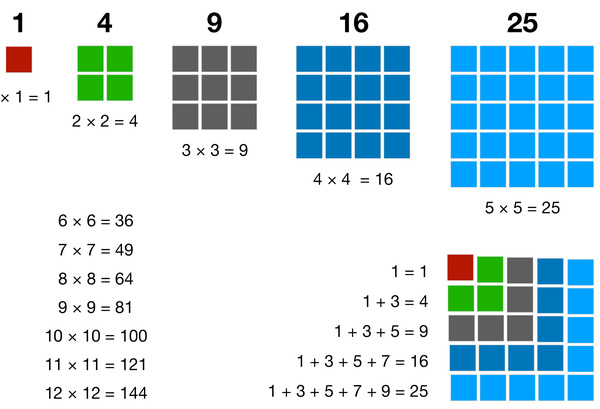# Number Blocks: Square NumbersA square number is the product of multiplying a number by itself. The concept of square numbers is introduced to kids until the fifth grade. Kids in sixth grade are expected to know the square numbers up to 12 x 12, and the square numbers of multiples of 10. They should recognize square numbers and use this knowledge to tackle further complex problems. Learning square numbers is very useful for working out the area of squares, and it also lays the foundation for studying cube and cube numbers.

This worksheet shows the first few square numbers illustrated by number blocks: 1, 4, 16, and 25. The square of a number is the same as finding the area of a square with side length of that number. There are infinitely many square numbers, and you can determine more: 36, 49, 64, 81, 100, and so on. Square numbers can also be generated by adding consecutive odd numbers. This worksheet is very useful to help kids understand the concept of square numbers, and make a connection between squaring and the area of a square.